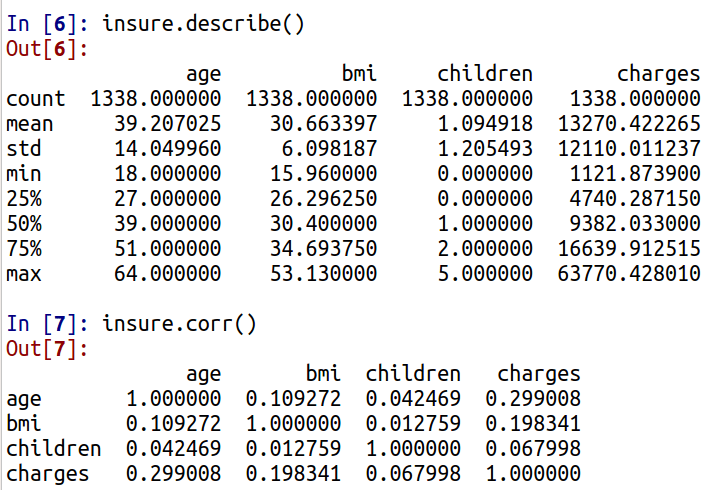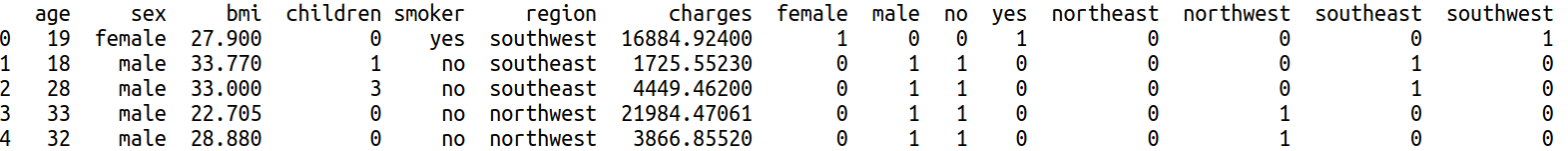# Multiple Regression in Python

In this post, we will go through the process of setting up and a regression model with a training and testing set using Python. We will use the insurance dataset from kaggle. Our goal will be to predict charges. In this analysis, the following steps will be performed.

1. Data preparation
2. Model training
3. model testing

Data Preparation

Below is a list of the modules we will need in order to complete the analysis

```import matplotlib.pyplot as plt
import pandas as pd
from sklearn import linear_model,model_selection, feature_selection,preprocessing
import statsmodels.formula.api as sm
from statsmodels.tools.eval_measures import mse
from sklearn.metrics import mean_squared_error```

After you download the dataset you need to load it and take a look at it. You will use the  .read_csv function from pandas to load the data and .head() function to look at the data. Below is the code and the output.

`insure=pd.read_csv('YOUR LOCATION HERE')`We need to create some dummy variables for sex, smoker, and region. We will address that in a moment, right now we will look at descriptive stats for our continuous variables. We will use the .describe() function for descriptive stats and the .corr() function to find the correlations.The descriptives are left for your own interpretation. As for the correlations, they are generally weak which is an indication that regression may be appropriate.

As mentioned earlier, we need to make dummy variables sex, smoker, and region in order to do the regression analysis. To complete this we need to do the following.

1. Use the pd.get_dummies function from pandas to create the dummy
2. Save the dummy variable in an object called ‘dummy’
3. Use the pd.concat function to add our new dummy variable to our ‘insure’ dataset
4. Repeat this three times

Below is the code for doing this

```dummy=pd.get_dummies(insure['sex'])
insure=pd.concat([insure,dummy],axis=1)
dummy=pd.get_dummies(insure['smoker'])
insure=pd.concat([insure,dummy],axis=1)
dummy=pd.get_dummies(insure['region'])
insure=pd.concat([insure,dummy],axis=1)The .get_dummies function requires the name of the dataframe and in the brackets the name of the variable to convert. The .concat function requires the name of the two datasets to combine as well the axis on which to perform it.

We now need to remove the original text variables from the dataset. In addition, we need to remove the y variable “charges” because this is the dependent variable.

```y = insure.charges
insure=insure.drop(['sex', 'smoker','region','charges'], axis=1)```

We can now move to model development.

Model Training

Are train and test sets are model with the model_selection.trainin_test_split function. We will do an 80-20 split of the data. Below is the code.

`X_train, X_test, y_train, y_test = model_selection.train_test_split(insure, y, test_size=0.2)`

In this single line of code, we create a train and test set of our independent variables and our dependent variable.

We can not run our regression analysis. This requires the use of the .OLS function from statsmodels module. Below is the code.

`answer=sm.OLS(y_train, add_constant(X_train)).fit()`

In the code above inside the parentheses, we put the dependent variable(y_train) and the independent variables (X_train). However, we had to use the function add_constant to get the intercept for the output. All of this information is then used inside the .fit() function to fit a model.

To see the output you need to use the .summary() function as shown below.

`answer.summary()`The assumption is that you know regression but our reading this post to learn python. Therefore, we will not go into great detail about the results. The r-square is strong, however, the region and gender are not statistically significant.

We will now move to model testing

Model Testing

Our goal here is to take the model that we developed and see how it does on other data. First, we need to predict values with the model we made with the new data. This is shown in the code below

`ypred=answer.predict(add_constant(X_test))`

We use the .predict() function for this action and we use the X_test data as well. With this information, we will calculate the mean squared error. This metric is useful for comparing models. We only made one model so it is not that useful in this situation. Below is the code and results.

```print(mse(ypred,y_test))
33678660.23480476```

For our final trick, we will make a scatterplot with the predicted and actual values of the test set. In addition, we will calculate the correlation of the predict values and test set values. This is an alternative metric for assessing a model.You can see the first two lines are for making the plot. Lines 3-4 are for making the correlation matrix and involves the .concat() function. The correlation is high at 0.86 which indicates the model is good at accurately predicting the values. THis is confirmed with the scatterplot which is almost a straight line.

Conclusion

IN this post we learned how to do a regression analysis in Python. We prepared the data, developed a model, and tested a model with an evaluation of it.